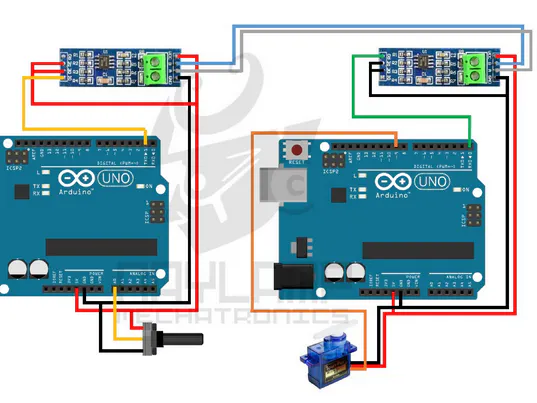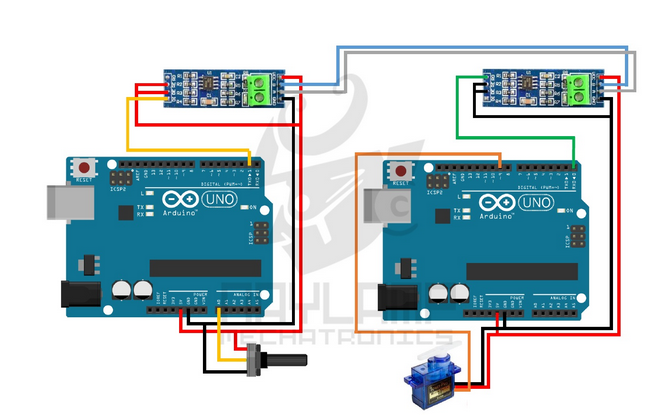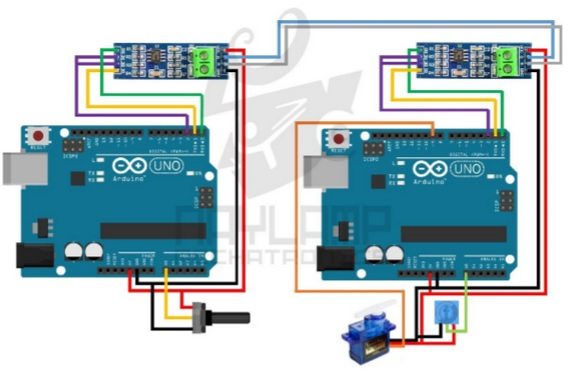Project showcaseModbus (RS-485) Using Arduino © GPL3+

We implement RS-485 protocol in communication between two Arduinos using MAX485 module.

• 89,392 views
• 16 respects

Apps and online services

RS-485 is one type of asynchronous serial communication protocol because there is no synchronizing clock signal transmitted along with the data. RS-485 uses differential signaling to transfer binary data from one device to another. Differential signaling worked by creating differential voltage using 5V positive and negative.This differential signaling method has the advantage in rejecting common mode noise.

RS-485 supports a maximum data transfer rate of 30 Mbps. RS-485 also supports many slaves with a single master. RS-485 protocol can have up to 32 devices connected.

In this project, we are going to implement RS-485 protocol in communication between two Arduinos using MAX485 module. This module uses 5V as operating voltage and has pinout configuration as shown in the following table.

RS-485 module as transmitter

In order to be used as a transmitter, RE pin and DE pin must be connected to 5V, and DI pin is connected to TX. Data is sent from Arduino TX pin to module DI pin, then data will be sent through AB.

In order to be used as a receiver, RE pin and DE pin must be connected to GND, and RO pin is connected to RX. Data received by AB will be sent to RO pin that is connected to Arduino RX pin so the data can be read by Arduino.

RS-485 can be implemented into three types of serial communication system: simplex, half-duplex, and full-duplex. But in this project, we are only implementing simplex and half-duplex.

Simplex communication between two Arduinos by RS485

Simplex communication is unidirectional communication (one sends data and the other only receives data), with one arduino behaving only as transmitter and the other only as receivers. In this experiment, the transmitter reads data from potentiometer to control the servo motor which is connected to the receiver.

The result is as shown in video below:

Half-duplex communication between two Arduinos by RS485

Half-duplex communication is two-way communication using one channel which we cannot receive and transfer data at the same time. Both arduino behave either as transmitter or receiver alternately, controlled by a GPIO pin (HIGH to enable as transmitter, LOW to enable as receiver). In this experiment, Arduino 1 not only reads data from potentiometer to control the servo motor connected to Arduino 2, but also receives data from sensor (represented as potentiometer) connected to Arduino 2, then turns on the built-in LED if the data has reached the threshold.

The result is as shown in video below:

Reference:

https://naylampmechatronics.com/blog/37_Comunicación-RS485-con-Arduino.html

Code

Peer 1 (Half Duplex)C/C++
Transmitter data frame
header + identifier + data value + footer

const int ledPin =  13;  // Built-in LED
const int EnTxPin =  2;  // HIGH:Transmitter, LOW:Receiver
void setup()
{
Serial.begin(9600);
Serial.setTimeout(100);
pinMode(ledPin, OUTPUT);
pinMode(EnTxPin, OUTPUT);
digitalWrite(ledPin, LOW);
digitalWrite(EnTxPin, HIGH);
}

void loop()
{
int rdata = analogRead(0); //data from potentiometer
int angle= map(rdata, 0, 1023, 0, 180);

//transmitter data packet
Serial.print("I"); //initiate data packet
Serial.print("S"); //code for servo
Serial.print(angle); //servo angle data
Serial.print("F"); //finish data packet
delay(50);

Serial.print("I"); //initiate data packet
Serial.print("L"); //code for sensor
Serial.print("F"); //finish data packet
Serial.flush();

if(Serial.find("i"))
{
int data=Serial.parseInt();
{
onLED(data);
}

}
digitalWrite(EnTxPin, HIGH); //RS485 as transmitter

}

void onLED(int data)
{
if(data>500)
digitalWrite(ledPin, HIGH);
else
digitalWrite(ledPin, LOW);
}
Peer 2 (Half Duplex)C/C++
Transmitter data frame
header + identifier + data value + footer

#include <Servo.h>
Servo myservo;
const int EnTxPin = 2;
void setup () {
Serial.begin (9600);
myservo.attach (9);
pinMode(EnTxPin, OUTPUT );
digitalWrite (EnTxPin, LOW );
}

void loop (){
if ( Serial.available ()){
if ( Serial.read () == 'I' ){
if (function == 'S' ){
int angle = Serial.parseInt ();
if ( Serial.read () == 'F' ){
if (angle <= 180) {
myservo.write (angle);
}
}
}
else if (function == 'L' ){
if ( Serial.read () == 'F' ){
digitalWrite (EnTxPin, HIGH ); //enable to transmit
Serial.print ( "i" );
Serial.print (val);
Serial.println ( "f" );
Serial.flush ();
digitalWrite (EnTxPin, LOW ); //enable to receive
}
}
}
}
delay (10);
}

#include <Servo.h>
Servo myservo;
const int EnTxPin = 2;
void setup () {
Serial.begin (9600);
myservo.attach (9);
pinMode(EnTxPin, OUTPUT );
digitalWrite (EnTxPin, LOW );
}

void loop (){
if ( Serial.available ()){
if ( Serial.read () == 'I' ){
if (function == 'S' ){
int angle = Serial.parseInt ();
if ( Serial.read () == 'F' ){
if (angle <= 180) {
myservo.write (angle);
}
}
}
else if (function == 'L' ){
if ( Serial.read () == 'F' ){
digitalWrite (EnTxPin, HIGH ); //enable to transmit
Serial.print ( "i" );
Serial.print (val);
Serial.println ( "f" );
Serial.flush ();
digitalWrite (EnTxPin, LOW ); //enable to receive
}
}
}
}
delay (10);
}
Transmitter (Simplex)C/C++
void setup()
{
Serial.begin(9600);
}

void loop()
{
byte angle= map(data, 0, 1023, 0, 180);
Serial.write(angle);
delay(50);
}
#include <Servo.h>

Servo myservo;

void setup()
{
Serial.begin(9600);
myservo.attach(9);
}

void loop()
{

if (Serial.available()) {
if(angle<=180)
{
myservo.write(angle);
}
}
}

Schematics

For transmitter (Arduino 1)
MAX485 Module -> Arduino
Vcc -> 5V
GND -> GND
RO -> -
RE -> 5V
DE -> 5V
DI -> TX

Potentiometer -> Arduino
Vcc -> 5V
GND -> GND
Output -> A0

MAX485 Module -> Arduino
Vcc -> 5V
GND -> GND
RO -> RX
RE -> GND
DE -> GND
DI -> -

Servo Motor -> Arduino
Vcc (Red) -> 5V
GND (Brown) -> GND
PWM (Orange) -> GPIO 9For both Arduino
MAX485 Module -> Arduino
Vcc -> 5V
GND -> GND
RO -> RX
RE -> GPIO 2
DE -> GPIO 2
DI -> TX

For Arduino 1
Potentiometer -> Arduino
Vcc -> 5V
GND -> GND
Output -> A0

For Arduino 2:
Servo Motor -> Arduino
Vcc (Red) -> 5V
GND (Brown) -> GND
PWM (Orange) -> GPIO 9

Potentiometer -> Arduino (Arduino 2)
Vcc -> 5V
GND -> GND
Output -> A0Created by

Maurizfa (13216008) / Arthur Jogy (13216037) / Agha Maretha (13216095)

Are you Maurizfa (13216008) / Arthur Jogy (13216037) / Agha Maretha (13216095)? Claim this project and add it to your profile.

May 17, 2020

Members who respect this project

See similar projects
you might like

CAN Bus Using Arduino

Project showcase by Maurizfa (13216008) / Arthur Jogy (13216037) / Agha Maretha (13216095)

• 51,387 views
• 15 respects

How to Use Modbus with Arduino

Project tutorial by hwhardsoft

• 48,919 views
• 27 respects

How to Communicate 3 or 32 Arduinos via RS-485

Project tutorial by Robotics Arduino

• 9,815 views
• 16 respects

Arduino Bluetooth Robot for Android Device

Project showcase by aip06

• 7,786 views
• 23 respects

ESP8266 ESP-01 Webserver

• 165,054 views